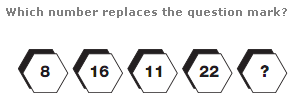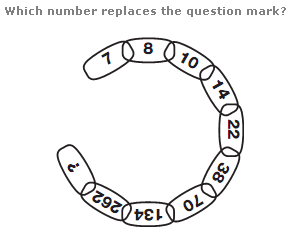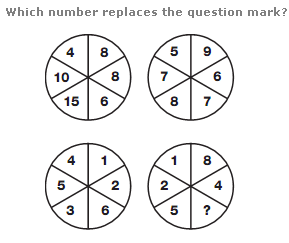# Puzzles - Number puzzles

### Exercise :: Number puzzlesAnswer : 17 Explanation : As you move to the right, double the first number to give the next one, then subtract 5. Repeat this sequence.Answer : 518 Explanation : As you move clockwise, subtract 3 from the first number and multiply by 2 to give the next one round.Answer : 1 Explanation : Numbers in the segments of the upper left hand circle equal the product of the numbers in corresponding segments of the lower 2 circles, and the numbers in the segments of the upper right hand circle equal the sum of the numbers in corresponding segments of the bottom two circles.